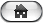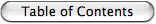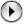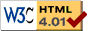HomeFirst - Prev - Contents - Next - LastDeutsch

Appendix A: Commodore VIC-20 BASIC V2

Here is a short summary of the commands for the built in Commodore VIC-20 BASIC V2. Note that this is not intended to be a tutorial, but just a quick reference to refresh long unused brain cells.
If you are looking for a BASIC tutorial, take a look at the original VIC-20 manual. It is available as etext at: http://project64.c64.org

In VIC-20 BASIC V2 there are only few types of variables:

• Float: This is the most frequently used type. Float-variables do not carry a type identifier.
Range +/- [2.94E-39 ... 1.70E+38] (+/- [2-128 ... 2127])
Precision: 32 bit mantisse (approx. 9.6 decimal digits) + 8 bit exponent
• Integer (16 Bit): This is a rarely used type. Integer variables carry a '%' at the end of their name (eg. X%). Note that all computations are performed with float precision and conversion to Integer is only performed when the value is assigned to a variable. Thus Integer math is SLOWER(!) than Float math.
• Boolean: Results are expressed using Integers. FALSE is represented by 0 (0x0000) and TRUE by -1 (0xFFFF) or any other non-zero value.
• Strings: String variables carry a '\$' at the end of their name (eg. X\$). Strings can be at most 255 characters long.

Only the first TWO (!) characters of a variable name are significant. Thus 'CO', 'COCOS' and 'COLLOSEUM' (but not 'C' and 'CA') are equivalent names for a single variable. On the other hand the type identifier is used to distinguish variables. Therefore 'CO', 'CO%' and 'CO\$' are three different variables.

Program Flow Control

FOR...TO...STEP...NEXT - The only real loop construct in BASIC
Syntax:
FOR <Var> = <Start> TO <End> [STEP <Size>]
<Loop Code>
NEXT [<Var>]
Examples:
FOR I=1 TO 5: PRINT I;: NEXT -> 1 2 3 4 5
FOR I=1 TO 5 STEP 2: PRINT I;: NEXT -> 1 3 5
FOR I=5 TO 1 STEP -2: PRINT I;: NEXT I -> 5 3 1
FOR I=3 TO 1: PRINT I;: NEXT -> 3 (!)
IF...THEN - Conditional Program Execution
Note: There is no such thing as ELSE or ENDIF.
Syntax:
IF <Condition> THEN <Statements> or
IF <Condition> GOTO <LineNr> or
IF <Condition> THEN <LineNr>
Example:
100 IF A < B THEN MN = A: GOTO 120
110 MN = B
120 ....
GOTO or GO TO - Unconditional Jump
Syntax:
GOTO <LineNr> or
GO TO <LineNr>
Note that it is not possible to use formal parameters to a subroutine. Everything must be done using global variables.
Syntax:
GOSUB <LineNr>
Example:
10 PRINT "Main Program"
20 GOSUB 100
30 PRINT "Back To Main"
40 GOSUB 100
50 PRINT "Once again Main"
60 END
100 PRINT "This is the Subroutine"
110 RETURN
RETURN - Return from Subroutine
Syntax:
RETURN
Example:
See GOSUB
ON...GOTO or ON...GOSUB - Multiway branch
Syntax:
ON <IntegerExpr> GOTO <LineNr1>, <LineNr2> ...
or ON <IntegerExpr> GOSUB <LineNr1>, <LineNr2> ...
Example:
ON X GOTO 100, 200, 300
This is equivalent to:
IF X = 1 THEN GOTO 100
IF X = 2 THEN GOTO 200
IF X = 3 THEN GOTO 300
DEF FN- Define a BASIC Function/Subroutine
Syntax:
DEF FN <Name>(<Param>) = <Single Line Expression>
Example:
DEF FN SI(X) = SIN(X)/X
FN SI(π/3) -> 0.826993343

Input/Output

GET - Read One Character from Standard Input without waiting
Syntax:
GET <VarName>
Example:
100 GET A\$: IF A\$ = "" THEN GOTO 100 -> Wait for any Key
INPUT - Get Data from Standard Input (usually Keyboard)
Syntax:
INPUT [<Prompt>;] <VarName> [, <VarName> ...]
Examples:
INPUT "Please Enter A, B and C"; A, B, C
INPUT A
PRINT - Write to Standard Output (usually Screen)
Syntax:
PRINT <Data> or
? <Data>
Examples:
PRINT "Hello World"
PRINT "Here", "are", "Tabs" -> Note the ','
PRINT "First Line"; -> Note the ';'
PRINT "Still the same line"
PRINT "Power"; 17+3
SPC - Advance the Cursor by a specific Number of Steps
Syntax:
SPC(<Cnt>)
Example:
SPC(6)
TAB- Advance the Cursor to a Specific Position
Syntax:
TAB(<Place>)
Example:
TAB(6)
POS - Current Cursor Position
Syntax:
POS(<Dummy>)

Files

Syntax:
LOAD <FileName> [, <Device> [, <SecondDev>]]
Example:
SAVE - Save a Program to Disk or Tape
Syntax:
SAVE <FileName> [, <Device> [, <SecondDev>]]
Example:
SAVE "SuperGame", 8 -> Save to Disk #8
To overwrite an existing file on a disk, prefix the filename with '@'
Example:
SAVE "@SuperGame",8 -> Save to Disk #8, overwriting an old file
VERIFY - Check if the Program in Memory and a Program on Disk or Tape are equal. Do not modify anything.
Syntax:
VERIFY <FileName> [, <Device> [, <SecondDev>]]
Example:
VERIFY "SuperGame", 8 -> Check SuperGame from Disk #8
VERIFY "*", 9 -> Check the first program from Disk #9
VERIFY "", 1 -> Check the first program from Tape #1
OPEN- Open a File
Syntax:
OPEN <FileID>, <Device> [, <SecondDev> [, <FNameMode>]]
The <SecondDev> is an optional integer in the range 0-15 with the following meaning: 0..Used for LOAD, 1..Used for SAVE, 2-14..Freely usable for User File Access, 15..Command/Error Channel.
<FNameMode> uses the format: "<FileName> [,<FileType> [,<AccessMode>]]" where <FileType> is one of P (Program), S (Sequential), L (Relative) or U (User) and <AccessMode> is one of R (Read), W (Write), A (Append) or the number of Bytes/Record for Relative Files.
Example:
OPEN 1, 4 -> Open a Output File to the Printer #4
OPEN 1, 8, 2, "My File,P,R" -> Open a Program for Reading
OPEN 1, 8, 2, "My File,S,W" -> Open a Sequential File for Writing
OPEN 1, 8, 2, "My File,L,"+CHR\$(40) -> Open a Relative File with 40 Bytes/Record
OPEN 1, 8, 15 -> Open the Disk Command/Error Channel
CLOSE - Close a File
Syntax:
CLOSE <FileID>
Example:
CLOSE 1
GET# - Read One Character from a File
Syntax:
GET# <FileID>, <VarName>
Example:
GET#1, A\$
Note that there is no space between 'GET' and '#'.
INPUT# - Get Data from a File
Syntax:
INPUT# <FileID>, <VarName> [, <VarName>...]
Example:
INPUT#1, EN\$, ER\$, TR\$, SC\$
Note that there is no space between 'INPUT' and '#'.
PRINT#
Syntax:
PRINT# <FileID>, <Data>
Example:
PRINT#1, "Power20"
Note that there is no space between 'PRINT' and '#'. Note also that ?# is not PRINT# also they look the same in a listing.
CMD - Redirect Standard Output (Input is not affected) and writes a Message to it
Syntax:
CMD <FileID> [, <Message>]
Example:
OPEN 1, 4 ; Open a File#1 on Printer#4
CMD 1 ; Make it the standard Output
PRINT "Whatever Output you want"
PRINT "More Output"
PRINT#1 ; Undo CMD 1
CLOSE 1
ST - Device Status (Built-In Variable)
ST = 0 .. Device Ok
Bit 6: 1 .. End of File
Bit 7: 1 .. Device Not Present
Syntax:
Example:
10 RESTORE
30 PRINT X\$;
40 S = 0
50 FOR I=1 TO 3
70 S = S + X
80 NEXT I
90 PRINT S
100 DATA "Power", 12, 34, -26
RESTORE - Set Pointer to Next DATA element to the first DATA statement in the program.
Syntax:
RESTORE
Example:
DATA- Store Static Data
Syntax:
DATA <Data> [, <Data>...]
Example:

Math Functions

LET - Assignment
Syntax:
LET <Variable> = <Value>
Example LET A = 6.25
Note the LET keyword is not necessary. <Variable> = <Value> is all that is needed. LET only slows things down -> Don't use it!
DIM - Array Declaration
Syntax:
DIM <Name>(<Size> [, <Size>...])
Example:
DIM A(7) -> An array of 8(!) elements indexed [0..7]
DIM B\$(4,5) -> An array of 30(!) strings
Usage of Elements: A(3) = 17 : B\$(2,3) = "Power20"
+, -, *, /, ^ - Arithmetic Operators
Example:
9 + 5 * (15 - 1) / 7 + 2^4 -> 35
<, <=, =, <>, >=, > - Comparison Operators
Examples:
3 <> 6 -> -1 (TRUE)
3 > 4 -> 0 (FALSE)
SIN - Sine (Argument in Radians)
Syntax:
SIN(<Value>)
Example:
SIN(π/3) -> 0.866025404
COS - Cosine (Argument in Radians)
Syntax:
COS(<Value>)
Example:
COS(π/3) -> 0.5
TAN - Tangent (Argument in Radians)
Syntax:
TAN(<Value>)
Example:
TAN(π/3) -> 1.73205081
ATN - Arcus Tangent (Result in [-π/2 .. π/2])
Syntax:
ATN(<Value>)
Example:
ATN(1) -> 0.785398163 ( = π/4)
EXP - Exponent (ex where e = 2.71828183...)
Syntax:
EXP(<Value>)
Example:
EXP(6.25) -> 518.012825
LOG - Natural Logarithm
Syntax:
LOG(<Value>)
Example:
LOG(6.25) -> 1.83258146
SQR - Square Root
Syntax:
SQR(<Value>)
Example:
SQR(6.25) -> 2.5
ABS - Absolute Value
Syntax:
ABS(<Value>)
Examples:
ABS(-6.25) -> 6.25
ABS(0) -> 0
ABS(6.25) -> 6.25
SGN - Sign
Syntax:
SGN(<Value>)
Examples:
SGN(-6.25) -> -1
SGN(0) -> 0
SGN(6.25) -> 1
INT - Integer (Truncate to greatest integer less or equal to Argument.)
Syntax:
INT(<Value>)
Examples:
INT(-6.25) -> -7 (!)
INT(-5) -> -5
INT(0) -> 0
INT(5) -> 5
INT(6.25) -> 6
RND - Random Number in [0.0 .. 1.0]
Syntax:
RND(<Seed>)
If (<Seed> < 0) the Random number generator is initialized
Examples:
RND(-625) -> 3.85114436E-06
RND(0) -> 0.464844882
RND(0) -> 0.0156260729

Logic & Binary Operators

Recall the encoding of Boolean Values:
FALSE <--> 0 (0x0000) and TRUE <--> -1 (0xFFFF) or any non-zero value

AND- Logical & Binary AND
Syntax:
<Expr> AND <Expr>
Examples:
A>5 AND X<=Y
12 AND 10 -> 8 (%1100 AND %1010 = %1000)
OR - Logical & Binary OR
Syntax:
<Expr> OR <Expr>
Examples:
A>5 OR X<=Y
12 OR 10 -> 14 (%1100 OR %1010 = %1110)
NOT- Logical & Binary NOT
Syntax:
NOT <Expr>
Examples:
NOT A>5
NOT 2 -> -3 (NOT \$0002 = \$FFFD)

Character & String Processing

+ - Concatenate Strings
Example:
"Pow" + "er20" -> "Power20"
<, <=, =, <>, >=, > - Comparison Operators
Examples:
"Power20" < "VIC-20" -> -1 (TRUE)
"Alpha" > "Omega" -> 0 (FALSE)
LEN - Stringlength
Syntax:
LEN(<String>)
Example:
LEN("Power20") -> 7
LEFT\$ - Left part of a string
Syntax:
LEFT\$(<String>, <Len>)
Example:
LEFT\$("Power20", 5) -> "Power"
RIGHT\$ - Right part of a string
Syntax:
RIGHT\$(<String>, <Len>)
Example:
RIGHT\$("Power20", 5) -> "wer20"
MID\$ - Middle part of a string
Syntax:
MID\$(<String>, <Start>, <Len>)
Example:
MID\$("Power20 for Macintosh", 13, 3) -> "Mac"
/* -- 123456789012345678901 -- */
STR\$ - Convert a Number into a String
Syntax:
STR\$(<Value>)
Examples:
STR\$(6.25) -> " 6.25"
STR\$(-6.25) -> "-6.25"
VAL - Convert a String to a Number
Syntax:
VAL(<String>)
Examples:
VAL("6.25") -> 6.25
VAL("6xx25") -> 6
VAL("x6x25") -> 0
ASC- ASCII code of the first character of a string
Syntax:
ASC(<String>)
Examples:
ASC("P") -> 80
ASC("Power20") -> 80
CHR\$ - Character with a specific ASCII code
Syntax:
CHR\$(<Value>)
Example:
CHR\$(80) -> "P"

Memory Access

PEEK - Read Byte from Memory
Syntax:
Example:
PEEK(36879) -> Current Background/Frame Color
POKE - Write Byte to Memory
Syntax:
Example:
POKE 36879, 47 -> Red Background and Yellow Frame
WAIT - Wait until a Byte in Memory has a specific value
Syntax:
WAIT will halt the program until ((PEEK(<Addr>) EXOR <Invert>) AND <Mask>) != 0
If <Invert> is not specified it is assumed to be 0.
Example:
WAIT 198, 255 -> Wait for a key in the key buffer.

Interface to Assembler Programs

SYS - System - Call a Assembler Program
Syntax:
The number of parameters depends on the actual program called.
USR- User Command
Syntax:
USR(<Param>)
Similar to SYS but the <Addr> is fixed to \$0310 and the first and only <Param> is already evaluated and stored in FloatAccu1 (FAC1) when the Assembler Program is called. Less flexible than SYS and thus rarely used.

Program Execution

RUN - Start the BASIC Program
Syntax:
RUN [<Line>]
If no <Line> is given, the program is started on its first line.
Example:
RUN
STOP- Stops program execution
Syntax:
STOP
STOP is similar to END, but prints the message BREAK IN <Line> when executed.
END - End program execution
Syntax:
END
CONT - Continue program execution
Syntax:
CONT
When program execution has interrupted by STOP, END or the Run/Stop key, the command CONT can be used to resume execution.

Miscellaneous

REM - Remark
Syntax:
REM <Text>
Example:
REM This line contains a comment
LIST - Display the listing of the current BASIC program
Syntax:
LIST [<Line> | <From>- | -<To> | <From>-<To>]
Without argument, the entire program is listed.
Examples:
LIST
LIST -40
LIST 100-200
NEW - Delete the current program and all variables from memory
Syntax:
NEW
CLR - Delete all variables
Syntax:
CLR
FRE - Free Memory
Syntax:
FRE(<Dummy>)
Example:
FRE(0) ->3581 (immediately after Power-on without RAM Expansion)
Returns the number of Bytes free for BASIC programs.
π - Pi = 3.14159265
TI - Timer Ticks since Power-On (1 Tick = 1/60 Second)
TI\$ - Timer since Power-On in Hour/Minute/Second Format
TI\$ (but not TI) can be assigned a value!
The accuracy of the Timer is very poor (>1% drift)Source: http://www.infinite-loop.at/Power20/Documentation/Power20-ReadMe/AA-VIC-20_BASIC.html Power20 Homepage: http://www.infinite-loop.at and http://www.salto.at - EMail: supportsaltoat © Roland Lieger, Goethegasse 39, A-2340 Mödling, Austria - Europe Last Changed: Feb. 29, 2008